Win up to 100% scholarship on Aakash BYJU'S JEE/NEET courses with ABNAT Win up to 100% scholarship on Aakash BYJU'S JEE/NEET courses with ABNAT

# JEE Main 2020 Paper with Solutions Physics 8th Jan Shift 2

JEE is an examination conducted for admission into various organisations across the country. The examination is conducted in two levels – JEE Main and JEE Advanced. The subjects for the engineering entrance tests are Mathematics, Physics and Chemistry. The questions are based on Class 11 and Class 12 syllabus. In this page, we have solved all the questions asked in JEE Main 2020 Physics (Shift 2, 8 January 2020)

### JEE Main 2020 Paper with Solutions Physics 8th Jan Shift 2

January 8 Shift 2 – Physics
1. A very long wire ABDMNDC is shown in figure carrying current 𝑖. AB and BC parts are straight, long and at right angle. At D wire forms a circular turn DMND of radius 𝑅. AB, BC are tangential to circular turn at N and D. Magnetic field at the centre of circle is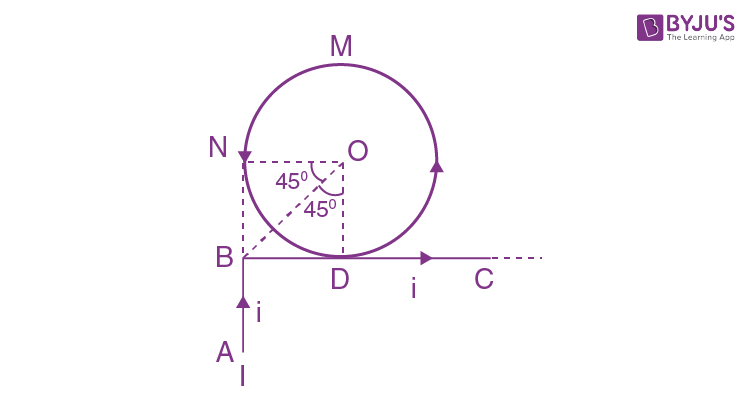a. 𝜇𝑜𝑖/2𝜋𝑅 [𝜋 −/1√2]
b. 𝜇𝑜𝑖/2𝜋𝑅[𝜋 + 1]
c. 𝜇𝑜𝑖/2𝑅
d. 𝜇𝑜𝑖/2𝜋𝑅 [𝜋 +1/√2]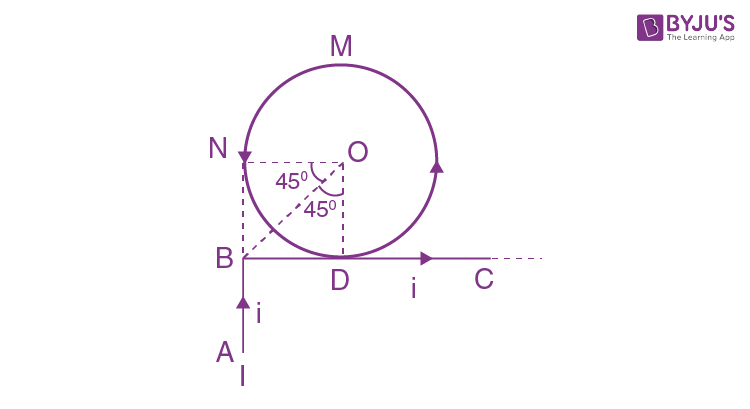To get magnetic field at O, we need to find magnetic field due to each current carrying part 1, 2, 3 and 4 individually Let’s take total magnetic field as 𝐵𝑇, then

$$\begin{array}{l}\vec{B_{T}}=\vec{B_{1}}+\vec{B_{2}}+\vec{B_{3}}+\vec{B_{4}}\end{array}$$

Since 2 and 4 are parts of same wire, hence

$$\begin{array}{l}\vec{B_{T}}\end{array}$$
= (𝜇𝑜𝑖/4𝜋𝑅)(sin 90° − sin 45°) (−
$$\begin{array}{l}\hat{k}\end{array}$$
) + 𝜇𝑜𝑖/2𝑅
$$\begin{array}{l}\hat{k}\end{array}$$
+ 𝜇𝑜𝑖/4𝜋𝑅(sin 90° + sin 45°)
$$\begin{array}{l}\hat{k}\end{array}$$

= (−𝜇𝑜𝑖/4𝜋𝑅) [1 −1/√2] +𝜇𝑜𝑖/2𝑅 +𝜇𝑜𝑖/4𝜋𝑅 [1 +1/√2]

$$\begin{array}{l}\hat{k}\end{array}$$

= (𝜇𝑜𝑖/4𝜋𝑅)[√2 + 2𝜋]

$$\begin{array}{l}\hat{k}\end{array}$$

= (𝜇𝑜𝑖/2𝜋𝑅) [1/√2+ 𝜋]

$$\begin{array}{l}\hat{k}\end{array}$$
$$\begin{array}{l}\hat{k}\end{array}$$
denotes that direction of magnetic field is in the plane coming out of the plane of

current.

2. A particle moves such that its position
$$\begin{array}{l}\vec{r(t)}=cos\omega t\hat{i}+sin\omega t\hat{j}\end{array}$$
, where 𝜔 is a constant and 𝑡 is time. Then which of the following statements is true for the velocity
$$\begin{array}{l}\vec{v}\end{array}$$
and acceleration
$$\begin{array}{l}\vec{a}\end{array}$$
(𝑡) of the particle?

a.
$$\begin{array}{l}\vec{v}\end{array}$$
and
$$\begin{array}{l}\vec{a}\end{array}$$
both are perpendicular to
$$\begin{array}{l}\vec{r}\end{array}$$

b.
$$\begin{array}{l}\vec{v}\end{array}$$
and
$$\begin{array}{l}\vec{r}\end{array}$$
both are parallel to
$$\begin{array}{l}\vec{r}\end{array}$$

c.
$$\begin{array}{l}\vec{v}\end{array}$$
is perpendicular to
$$\begin{array}{l}\vec{r}\end{array}$$
and
$$\begin{array}{l}\vec{a}\end{array}$$
is directed away from the origin

d.
$$\begin{array}{l}\vec{v}\end{array}$$
is perpendicular to
$$\begin{array}{l}\vec{r}\end{array}$$
and
$$\begin{array}{l}\vec{a}\end{array}$$
is directed towards the origin

$$\begin{array}{l}\vec{r(t)}=cos\omega t\hat{i}+sin\omega t\hat{j}\end{array}$$
$$\begin{array}{l}\vec{r(t)}=(dr/dt)= \omega[-sin\omega t\hat{i}+cos\omega \hat{j}\end{array}$$
$$\begin{array}{l}\vec{a}=(d\vec{v}/dt)=-\omega^{2}[cos\omega t\hat{i}+sin\omega \hat{j}]\end{array}$$
$$\begin{array}{l}\vec{a}=(d\vec{v}/dt)=-\omega^{2}\vec{r}\end{array}$$

Since there is negative sign in acceleration, this means that acceleration is in opposite direction of r⃗. For velocity direction we can take dot product of v and r.

$$\begin{array}{l}\vec{v}.\vec{r}=\omega (-sin\omega t\hat{i}+cos\omega t\hat{j} )(cos\omega t\hat{i}+sin\omega t\hat{j})\end{array}$$

= 𝜔[−sin 𝜔𝑡 cos 𝜔𝑡 + cos 𝜔𝑡 sin 𝜔𝑡] = 0

This implies that

$$\begin{array}{l}\vec{v}\end{array}$$
is perpendicular to
$$\begin{array}{l}\vec{r}\end{array}$$

3. Consider two charged metallic spheres 𝑆1 and 𝑆2 of radii 𝑟1 and 𝑟2, respectively. The electric fields 𝐸1(on 𝑆1) and 𝐸2(on 𝑆2) on their surfaces are such that 𝐸1/𝐸2 = 𝑟1/ 𝑟2. Then the ratio 𝑉1(on 𝑆1)/𝑉2(on 𝑆2) of the electrostatic potentials on each sphere is
a. 𝑟1/𝑟2
b. (𝑟1/𝑟2)2
c. 𝑟2/𝑟1
d. (𝑟1/𝑟2)3

𝐸1/𝐸2 = 𝑟1/𝑟2

𝑉1/𝑉2 =𝐸1𝑟1/𝐸2𝑟2 = (𝑟1/𝑟2) × (𝑟1/𝑟2)

= (𝑟1/𝑟2)2

4. A transverse wave travels on a taut steel wire with a velocity of 𝑉 when tension in it is 2.06 × 104 𝑁. When the tension is changed to 𝑇, the velocity changed to 𝑉/2. The value of 𝑇 is close to
a. 30.5 × 104 𝑁
b. 2.50 × 104 𝑁
c. 10.2 × 102 𝑁
d. 5.15 × 103 𝑁

𝑉 ∝ √𝑇

𝑉1 /𝑉2 = √ 𝑇1/ 𝑇2

⇒ 2𝑉/ 𝑉 = √ (2.06 × 104 ) /𝑇

⇒ 𝑇 = 2.06 × 104/4 𝑁 = 5.15 × 103 N

5. A particle of mass 𝑚 is dropped from a height ℎ above the ground. At the same time another particle of the same mass is thrown vertically upwards from the ground with a speed of √2𝑔ℎ. If they collide head-on completely inelastically, the time taken for the combined mass to reach the ground, in units of √ ℎ/𝑔 is
a. √3/2
b. √1/2
c. 1/2
d. √3/4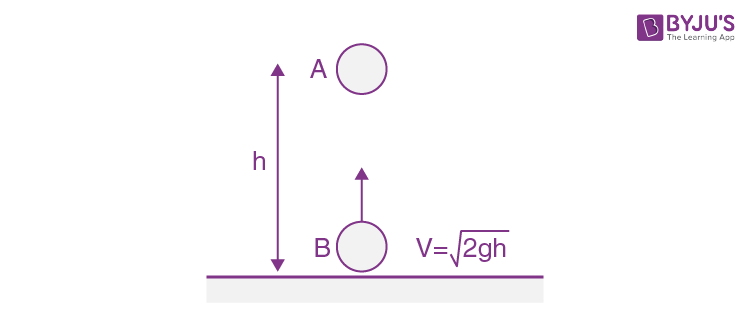Time taken for the collision 𝑡1 =ℎ/√2𝑔ℎ

After 𝑡1

𝑉𝐴 = 0 – 𝑔𝑡1 = −√𝑔ℎ/2

And 𝑉𝐵 = √2𝑔ℎ – 𝑔𝑡1 = √𝑔ℎ [√2 –1/√2]

At the time of collision

$$\begin{array}{l}\vec{P_{i}}=\vec{P_{f}}\end{array}$$

Hence,

$$\begin{array}{l}m\vec{V_{A}}+m\vec{V_{B}}=m\vec{V_{f}}\end{array}$$

Therefore, − (√𝑔ℎ/2) + (√𝑔ℎ [√2–1/√2]) =

$$\begin{array}{l}2\vec{V_{f}}\end{array}$$

𝑉𝑓 = 0

And height from the ground = ℎ − (1/2)𝑔 𝑡12 = ℎ – ℎ/4 =3ℎ/4

So, time taken to reach ground after collision = √2 ×[(3ℎ/4)/𝑔]

= √3ℎ/2𝑔

6. A Carnot engine having an efficiency of 1/10 is being used as a refrigerator. If the work done on the refrigerator is 10 J, the amount of heat absorbed from the reservoir at lower temperature is
a. 99 J
b. 90 J
c. 1 J
d. 100 J

For Carnot engine using as refrigerator

Work done on engine is given by

𝑊 = 𝑄1 − 𝑄2 … (1)

Where, 𝑄1 is heat rejected to the reservoir at higher temperature and 𝑄2 is the heat absorbed from the reservoir at lower temperature.

It is given = 1/10

𝜂 = 1 − 𝑄2/𝑄1

⟹ 𝑄2/ 𝑄1 = 9/10 ….. (2)

We are given, 𝑊 = 10 J

Therefore, from equations (1) and (2), 𝑄2 = 10/((10/9)– 1)

⇒ 𝑄2 = 90 J

7. Two liquids of density 𝜌1 and 𝜌2 (𝜌2 = 2𝜌1) are filled up behind a square wall of side 10 𝑚 as shown in figure. Each liquid has a height of 5 𝑚. The ratio of forces due to these liquids exerted on the upper part MN to that at the lower part NO is (Assume that the liquids are not mixing)a. 2/3
b. 1/2
c. 1/4
d. 1/ 3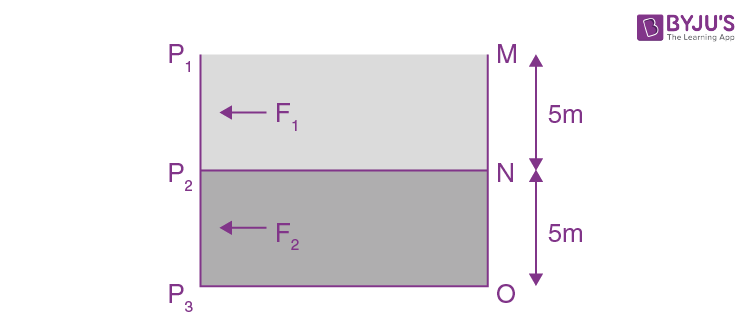The net force exerted on the wall by one type of liquid will be average value of pressure due to that liquid multiplied by the area of the wall.

Here, since the pressure due a liquid of uniform density varies linearly with depth, its average will be just the mean value of pressure at the top and pressure at the bottom.

So,

𝑃1 = 0

𝑃2 = 𝜌𝑔 × 5

𝑃3 = 5𝜌𝑔 + 2𝜌𝑔 × 5

𝐹1 = [(𝑃1 + 𝑃2)/2]𝐴

𝐹2 = [(𝑃2 + 𝑃3)/2) 𝐴

So,

𝐹1/𝐹2 = ¼

8. As shown in figure, when a spherical cavity (centered at O) of radius 1 m is cut out of a uniform sphere of radius 𝑅 (centered at C ), the center of mass of remaining (shaded) part of sphere is shown by COM, i.e. on the surface of the cavity. R can be determined by the equationa. (𝑅 2 + 𝑅 + 1)(2 − 𝑅) = 1
b. (𝑅 2 − 𝑅 − 1)(2 − 𝑅) = 1
c. (𝑅 2 − 𝑅 + 1)(2 − 𝑅) = 1
d. (𝑅 2 + 𝑅 − 1)(2 − 𝑅) = 1Let 𝑀 be the mass of the sphere and 𝑀’ be the mass of the cavity.

Mass of the remaining part of the sphere = 𝑀 – 𝑀’

Mass moments of the cavity and the remaining part of sphere about the original COM should add up to zero.

(𝑀 − 𝑀′ )(2 − 𝑅) − 𝑀′ (𝑅 − 1) = 0

(Mass of the cavity to be taken negative)

⇒ (4/3) 𝜋(𝑅 3 − 13 )𝜌𝑔 (2 − 𝑅) = (4/3) 𝜋(1) 3𝜌𝑔(𝑅 − 1)

⇒ (𝑅 3 − 1 3)(2 − 𝑅) = (1 3 )(𝑅 − 1)

⇒ (𝑅 2 + 𝑅 + 1)(𝑅 − 1)(2 − 𝑅) = (𝑅 − 1)

(using identity) ⇒ (𝑅 2 + 𝑅 + 1)(2 − 𝑅) = 1

9. A particle of mass 𝑚 and charge 𝑞 is released from rest in uniform electric field. If there is no other force on the particle, the dependence of its speed V on the distance x travelled by it is correctly given by (graphs are schematic and not drawn to scale)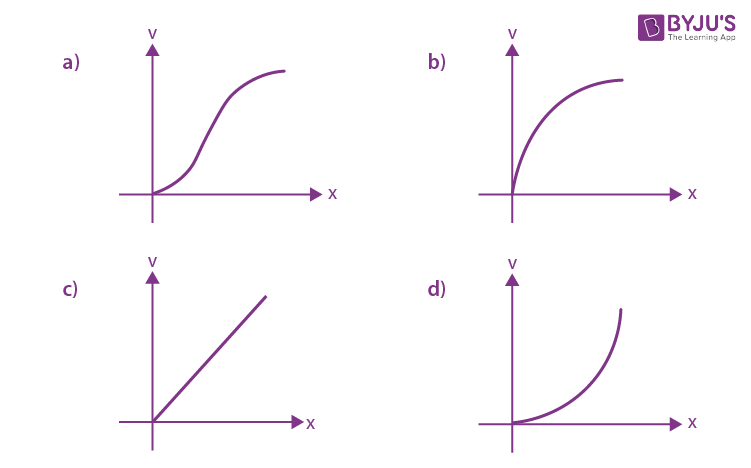Applying work energy theorem, 𝑞𝐸𝑥 = (1/2) 𝑚𝑣 2

𝑣 2 ∝ 𝑥

Hence, solution will be option (b)

10. A galvanometer having a coil resistance 100 Ω gives a full scale deflection when a current of 1 mA is passed through it. What is the value of the resistance which can convert this galvanometer into voltmeter giving full scale deflection for a potential difference of 10 V? In full scale deflection, current in galvanometer of resistance is 1 𝑚𝐴. Resistance required in series to convert it into voltmeter of range 10 𝑉.
a. 7.9 𝑘Ω
b. 9.9 𝑘Ω
c. 8.9 𝑘Ω
d. 10 𝑘Ω

𝑉0 = 𝑖𝑔 𝑅𝑔 = 0.1 𝑣

𝑣 = 10 𝑉

𝑅 = 𝑅𝑔 ((𝑣/𝑣𝑔) − 1) = 100 × 99 = 9.9 𝑘Ω

11. Consider a mixture of 𝑛 moles of helium gas and 2𝑛 moles of oxygen gas (molecules taken to be rigid) as an ideal gas. Its (𝐶𝑝/𝐶𝑣) value will be
a. 67/45
b. 40/27
c. 19/13
d. 23/15

Using formula 𝐶𝑣𝑚𝑖𝑥 = (𝑛1𝐶𝑣1 + 𝑛2𝐶𝑣2)/(𝑛1 + 𝑛2)

𝐶𝑣𝑚𝑖𝑥 = (𝑛× (3𝑅/2) +2𝑛× (5𝑅/2))/3𝑛 = 13𝑅/6

(∵ 𝐻𝑒 is monoatomic and 𝑂2 is diatomic)

𝐶𝑝𝑚𝑖𝑥 = 𝐶𝑣𝑚𝑖𝑥 + 𝑅 = 19𝑅/6

∴ 𝛾𝑚𝑖𝑥 = 𝐶𝑝𝑚𝑖𝑥 /𝐶𝑣𝑚𝑖𝑥 = 19/13

12. A uniform sphere of mass 500 𝑔𝑚 rolls without slipping on a plane horizontal surface with its centre moving at a speed of 5 𝑐m/𝑠. Its kinetic energy is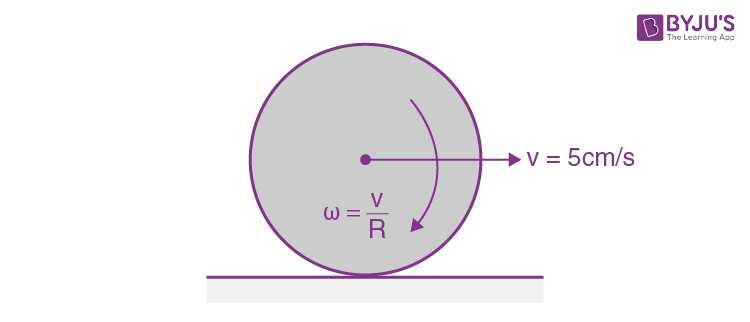a. 8.75 × 10−4 𝐽
b. 6.25 × 10−4 𝐽
c. 8.75 × 10−3 𝐽
d. 1.13 × 10−3 𝐽

Total K.E. = Translational K.E + Rotational K.E.

= 1/2 𝑚𝑣 2 + 1/2 2𝐼

= 1/2 𝑚𝑣 2 (1 + 𝑘 2/𝑅2 )

= 1/2 × 1/2 × ( 5/100) 2 (1 + (2/5) ) = 8.75 × 10−4 J

13. A capacitor is made of two square plates each of side ‘𝑎’ making a very small angle 𝛼 between them, as shown in figure. The capacitance will be close to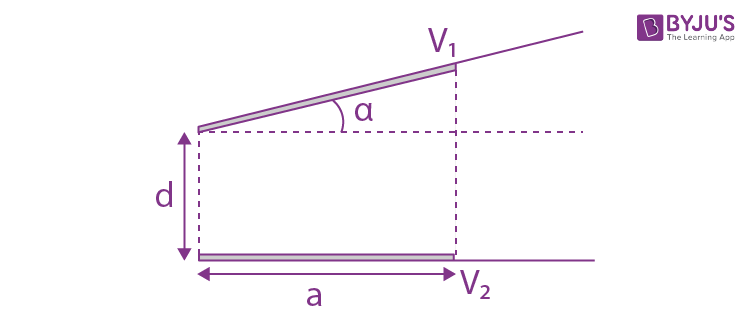a. ε0 𝑎2/𝑑 (1 – (𝛼𝑎/2𝑑))
b. ε0 𝑎2 /𝑑 (1 – (3𝛼𝑎/2𝑑))
c. ε0 𝑎2 𝑑 (1 + (𝛼𝑎/𝑑))
d. ε0 𝑎2 𝑑 (1 – (𝛼𝑎/4𝑑))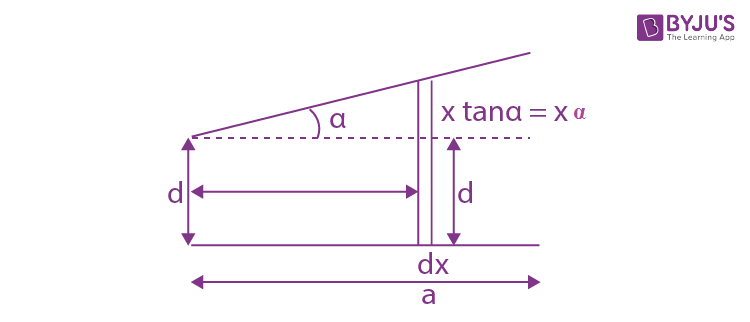Let 𝑑𝐶 be the capacitance of the element of thickness 𝑑𝑥

𝑑𝑐 = 𝜀0𝑎𝑑/(𝑑 + 𝛼𝑥)

These are effectively in parallel combination

So, 𝐶 = ∫ 𝑑𝑐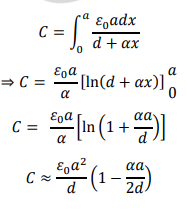14. In a double-slit experiment, at a certain point on the screen the path difference between the two interfering waves is 1 8 𝑡ℎ of a wavelength. The ratio of the intensity of light at that point to that at the centre of a bright fringe is
a. 0.568
b. 0.853
c. 0.672
d. 0.760

In YDSE, the intensity at a point on the screen varies with the phase difference between the interfering light waves as:

𝐼 = 𝐼0 𝑐𝑜𝑠2 ( ∆∅ 2 )

Here, ∆∅ = phase difference between the interfering waves

𝐼0 = maximum intensity on the screen

𝐼/𝐼0 = 𝑐𝑜𝑠2 [(2𝜋 𝜆 × ∆𝑥)/ 2 ] = cos2 ( 𝜋/8 )

𝐼/ 𝐼0 = 0.853

15. As shown in figure, a battery of emf 𝜀 is connected to an inductor 𝐿 and resistance 𝑅 in series. The switch is closed at 𝑡 = 0. The total charge that flows from the battery, between 𝑡 = 0 and 𝑡 = 𝑡𝑐 (𝑡𝑐 is the time constant of the circuit) is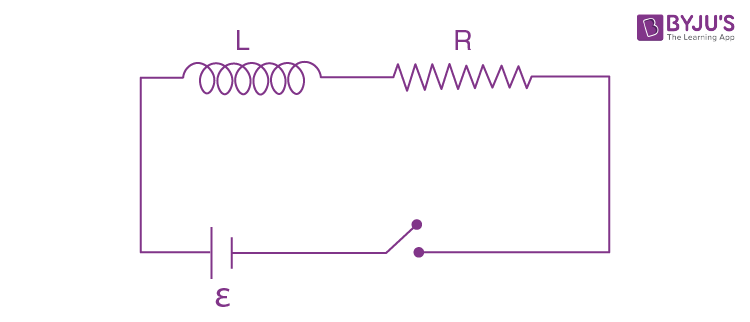a. ε𝐿/𝑒𝑅2
b. ε𝑅 𝑒𝐿2
c. ε𝐿/𝑅2 (1 – (1/𝑒) )
d. ε𝐿/𝑅2

This is standard 𝐿 − 𝑅 growth of current circuit.

𝑖(𝑡) = (𝜀/𝑅) 𝑒 − 𝑡 𝑇𝑐

Substituting in the integral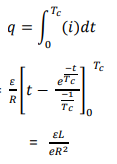16. A ball is dropped from the top of a 100 𝑚 high tower on a planet. In the last 1/2 𝑠 before hitting the ground, it covers a distance of 19 𝑚. Acceleration due to gravity (in 𝑚𝑠 −2) near the surface on that planet is Solution: 𝑔 = 8 𝑚/𝑠 2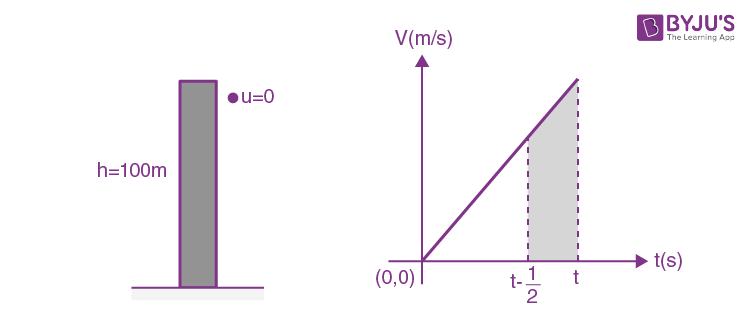Since the radius of planet is much larger than100 𝑚, it’s a uniformly accelerated motion. So, Trapezium’s area

𝑠 = [𝑔([𝑡− (1/2) + 𝑡]/2]× (1/2) = 19—- (i)

(1/2) 𝑔𝑡 2= 100——- (ii)

Solving equations (i) and (ii), we get 𝑔 = 8 𝑚/𝑠2

17. An object is gradually moving away from the focal point of a concave mirror along the axis of the mirror. The graphical representation of the magnitude of linear magnification (m) versus distance of the object from the mirror (x) is correctly given by (Graphs are drawn schematically and are not to scale)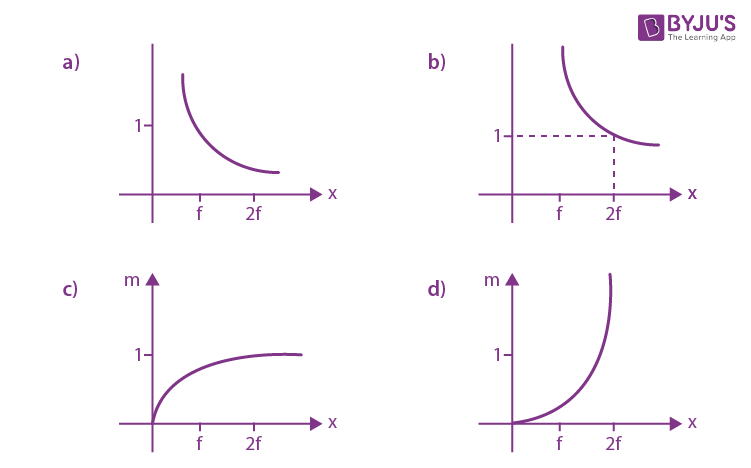Magnitude of linear magnification, m= |− 𝑣/𝑥 |

From mirror formula,

(1/𝑣) + (1/𝑥) = 1/𝑓

Multiplying the whole equation by 𝑢, we get 𝑣

𝑥 = 𝑓/(𝑥 – 𝑓)

Using the above equation in magnification formula,

𝑚 = |− 𝑣/𝑥 | = | 𝑓/(𝑓 – 𝑥) |

For 𝑥 = 2𝑓, 𝑚 = 1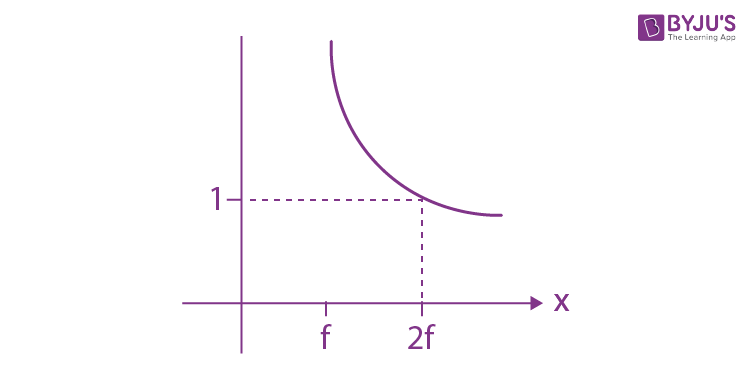18. A simple pendulum is being used to determine the value of gravitational acceleration 𝑔 at a certain place. The length of the pendulum is 25.0 𝑐𝑚 and a stop watch with 1 sec resolution measures the time taken for 40 oscillations to be 50 sec. The accuracy in 𝑔
a. 5.40%
b. 3.40%
c. 4.40%
d. 2.4%

𝑇 = 2𝜋√ 𝐿/𝑔

𝑔 = 𝐿. ( 2𝜋 𝑇 )2

(∆𝑔/𝑔) = 2 (∆𝑇/𝑇) + (∆𝐿 𝐿)

2( 1/50) + 0.1/25 = 4.40%

19. An electron (mass 𝑚) with initial velocity
$$\begin{array}{l}\vec{v}=v_{0}\hat{i}+v_{0}\hat{j}\end{array}$$
is in an electric field
$$\begin{array}{l}\vec{E}=-E_{0}\hat{k}\end{array}$$
. If 𝜆0 is initial de-Broglie wavelength of electron, its de-Broglie wavelength at time 𝑡 is given by

a.
$$\begin{array}{l}\lambda =\frac{\lambda _{0}}{\sqrt{1+\frac{E_{0}^{2}e^{2}}{m^{2}v_{0}^{2}}t^{2}}}\end{array}$$

b.
$$\begin{array}{l}\lambda =\frac{\lambda _{0}\sqrt{2}}{\sqrt{1+\frac{E_{0}^{2}e^{2}}{m^{2}v_{0}^{2}}t^{2}}}\end{array}$$

c.
$$\begin{array}{l}\lambda =\frac{\lambda _{0}}{\sqrt{1+\frac{E_{0}^{2}e^{2}}{2m^{2}v_{0}^{2}}t^{2}}}\end{array}$$

d.
$$\begin{array}{l}\lambda =\frac{\lambda _{0}}{\sqrt{2+\frac{E_{0}^{2}e^{2}}{m^{2}v_{0}^{2}}t^{2}}}\end{array}$$

Momentum of an electron, 𝑝 = 𝑚𝑣 = ℎ/𝜆

Initially 𝑚(√2𝑣0) = ℎ/𝜆0

Velocity as a function of time=𝑣0𝑖̂+ 𝑣0𝑗̂+ (𝑒𝐸0/𝑚)𝑡 𝑘̂

So, wavelength

$$\begin{array}{l}\lambda =\frac{h}{m\sqrt{2v_{0}^{2}+\frac{E_{0}^{2}e^{2}}{m^{2}}t^{2}}}\end{array}$$
$$\begin{array}{l}\lambda =\frac{\lambda _{0}}{\sqrt{1+\frac{E_{0}^{2}e^{2}}{m^{2}v_{0}^{2}}t^{2}}}\end{array}$$

20. In the given circuit, value of 𝑌 is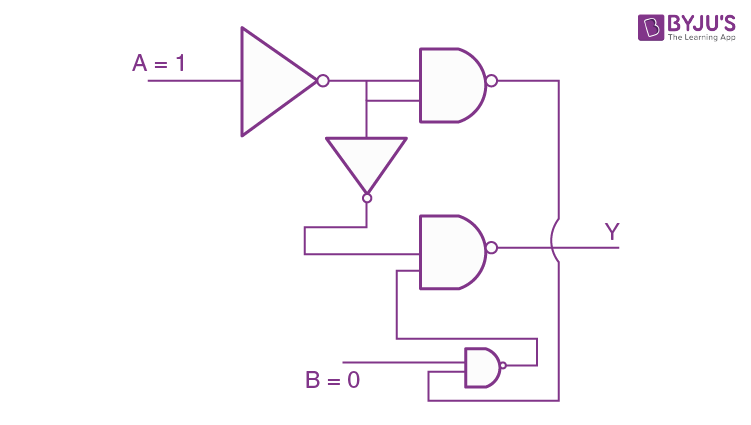a. 1
b. 0
c. Will not execute
d. Toggles between 0 and 1

$$\begin{array}{l}Y = \bar{\bar{AB}.A}\end{array}$$
$$\begin{array}{l}Y=\bar{\bar{AB}}+\bar{A}\end{array}$$

= 𝐴𝐵 + 𝐴̅

𝑌 = 0 + 0 = 0

21. The first member of Balmer series of hydrogen atom has a wavelength of 6561 Å. The wavelength of the second member of the Balmer series (in nm) is

$$\begin{array}{l}\Delta E = \frac{hC}{\lambda } = \Delta E_{o}\left [ \frac{1}{4} – \frac{1}{9} \right ]\end{array}$$
….i

$$\begin{array}{l}So, \frac{hC}{\lambda’} = \Delta E_{o}\left [ \frac{1}{4} – \frac{1}{16} \right ]\end{array}$$
….ii

$$\begin{array}{l}\Rightarrow \frac{\lambda’}{\lambda} = \frac{5\times 16}{9\times4 \times3 }\end{array}$$
$$\begin{array}{l}\Rightarrow \lambda’ = \frac{5\times4\times 656.1}{9\times3 } = 486\: nm\end{array}$$

22. A plane electromagnetic wave of frequency 25 GHz is propagating in vacuum along the zdirection. At a particular point in space and time, the magnetic field is given by
$$\begin{array}{l}\vec{B}=5 \times 10^{-8}\hat{j}T\end{array}$$
. The corresponding electric field is given by
$$\begin{array}{l}\vec{E}\end{array}$$
is (speed of light 𝑐 = 3 × 10 8 𝑚/𝑠)

a. 1.66 × 10−16𝑖̂ V/m
b. −1.66 × 10−16𝑖̂ V/m
c. 15𝑖̂ V/m
d. −15𝑖̂ V/m

|𝐸|/ |𝐵| = 𝑐

|𝐸| = |𝐵| × 𝑐

Given,

$$\begin{array}{l}\vec{B}=5 \times 10^{-8}\hat{j}T\end{array}$$
. and 𝐶 = 3 × 108 𝑚 𝑠 Therefore, |𝐸| = 15 𝑣𝑜𝑙𝑡 𝑚

Direction of by

$$\begin{array}{l}\vec{E}\end{array}$$
will be perpendicular to
$$\begin{array}{l}\vec{B}\end{array}$$
and
$$\begin{array}{l}\vec{E}\times \vec{B}\end{array}$$
gives direction of propagation of wave which is in z-direction (𝑘̂). Therefore, 𝐸⃗ will be along positive x-direction (𝑖̂).

Hence,

$$\begin{array}{l}\vec{E}\end{array}$$
= 15𝑖̂ V/m

23. Three containers 𝐶1, 𝐶2 and 𝐶3 have water at different temperatures. The table below shows the final temperature T when different amounts of water (given in liters) are taken from each container and mixed (assume no loss of heat during the process)

 𝐶1 𝐶2 𝐶3 𝑇(oC) 1 𝑙 2 𝑙 — 60 — 1 𝑙 2 𝑙 30 2 𝑙 — 1 𝑙 60 1 𝑙 1 𝑙 1 𝑙

The value of 𝜃 (in ℃ to the nearest integer) is

Since, all the containers have same material, specific heat capacity is the same for all.

𝑉1𝜃1 + 𝑉2𝜃2 = (𝑉1+𝑉2)𝜃𝑓

From second row of table,

1𝜃1 + 2𝜃2 = (1 + 2)60

𝜃1 + 2𝜃2 = 180…………… (1)

From third row of table,

0 × 𝜃1 + 1 × 𝜃2 + 2 × 𝜃3 = (1 + 2)30

𝜃2 + 2𝜃3 = 90…………… (2)

From fourth row of table, 2𝜃1 + 𝜃3 = (1 + 2)60

2𝜃1 + 𝜃3 = 180…………… (3)

From fifth row of table, 𝜃1 + 𝜃2 + 𝜃3 = (1 + 1 + 1)𝜃

𝜃1 + 𝜃2 + 𝜃3 = 3𝜃………… (4)

From equation. (1) + (2) + (3)

3𝜃1 + 3𝜃2 + 3𝜃3 = 450

Thus, 𝜃1 + 𝜃2 + 𝜃3 = 150

From (4) equation 150 = 3𝜃

So, 𝜃 = 500C

24. An asteroid is moving directly towards the centre of the earth. When at a distance of 10R (R is the radius of the earth) from the earth’s centre, it has a speed of 12 𝑘𝑚/𝑠. Neglecting the effect of earth’s atmosphere, what will be the speed of the asteroid when it hits the surface of the earth (escape velocity from the earth is 12 𝑘𝑚/𝑠 )? Give your answer to the nearest integer in 𝑘𝑚/𝑠

Taking, asteroid and earth as an isolated system conserving Total energy.

𝐾𝐸𝑖 + 𝑃𝐸𝑖 = 𝐾𝐸𝑓 + 𝑃𝐸𝑓

(1/2) 𝑚𝑢0 2 + (− 𝐺𝑀𝑚/10𝑅 ) = (1/2) 𝑚𝑣 2 + (− 𝐺𝑀𝑚/𝑅 )

𝑣 2 = 𝑢0 2 + 2𝐺𝑀 𝑅 [1 – (1/10)]

$$\begin{array}{l}v= \sqrt{u_{0}^{2}+\frac{9}{5}\frac{GM}{R}}\end{array}$$

Since, escape velocity from surface of earth is 11.2 𝑘𝑚/𝑠𝑒𝑐2 = √ 2𝐺𝑀 𝑅

$$\begin{array}{l}v= \sqrt{12^{2}+\frac{9}{5}\frac{(11.5)^{2}}{2}}\end{array}$$

= √256.9 ≈ 16 𝑘𝑚/𝑠

25. The series combination of two batteries both of the same emf 10 𝑉, but different internal resistance of 20 Ω and 5 Ω , is connected to the parallel combination of two resistors 30 Ω and 𝑅 Ω. The voltage difference across the battery of internal resistance 20 Ω is zero, the value of 𝑅 (in Ω) is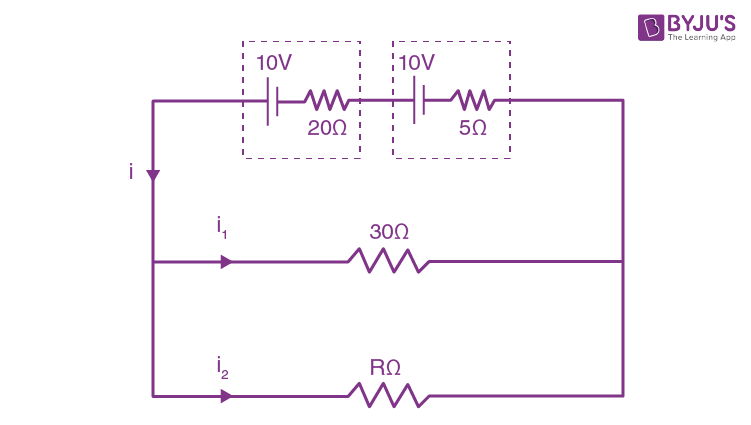If 𝑉1 and 𝑉2 are terminal voltage across the two batteries.

𝑉1 = 0

𝑉1 = 𝜀1 − 𝑖. 𝑟1

0 = 10 − 𝑖 × 20

𝑖 = 0.5 𝐴

𝑉2 = 10 − 0.5 × 5

𝑉2 = 7.5 𝑉

0.5 = (7.5/30) + (7.5/ 𝑥)

7.5/𝑥 = 0.25

𝑥 = 30